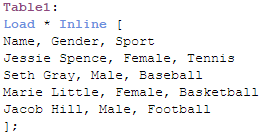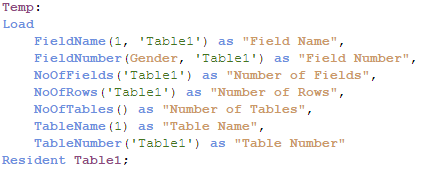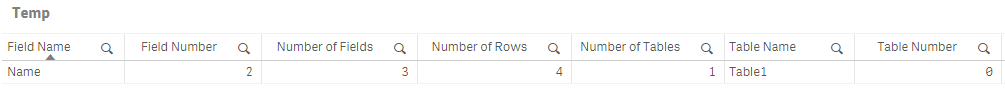Qlik Design Blog

All about product and Qlik solutions: scripting, data modeling, visual design, extensions, best practices, etc.Employee

Table Functions

Today, I am going to blog about Table functions.  I came across these functions when I was exploring the various ways I can determine if a table exists in the data model.  The table functions I will review today include:

• FieldName
• FieldNumber
• NoOfFields
• NoOfRows
• NoOfTables
• TableName
• TableNumber

These functions provide information about the current table being loaded or specified in the function.  All of these functions should be used in the script but the NoOfRows function can also be used in a chart expression.  Let’s take a closer look at these functions but first let’s load a table that we can work with.Now load the script to illustrate the functions:Here are the results of the Temp table loaded above:While the function names give you a good idea on what they are used for, let’s take a more detailed look at each one of them.

FieldName

The FieldName function takes two parameters, field number and table name, and returns the name of the respective field.  Based on the script above, the FieldName function will return Name since it is field 1 in the Table1 table.  Note, field numbers start with 1, not 0.

FieldNumber

The FieldNumber function takes two parameters, field name and table name, and returns the number of the specified field in the table.  In this case, Gender has a field number of 2 in Table1 so 2 is returned by the FieldNumber function.

NoOfFields

The NoOfFields function takes one parameter, table name, and returns the number of fields in the table that was specified.  The table name parameter must be for a table that is already loaded in the data model.  It cannot be for the table that is currently being loaded.  For example, in the script above, the table Temp could not be used in the NoOfFields function since that is the table being loaded.

NoOfRows

The NoOfRows function also takes one parameter, table name, and returns the number of rows in the specified table.  Like the NoOfFields function, this function must be used for a table that was previously loaded.

NoOfTables

The NoOfTables function does not take any parameters and returns the number of tables that have previously been loaded in the data model.  Based on the script above, the NoOfTables function will return 1 for the Table1 table.  Note that it does not include the Temp table that is being loaded when this function executes.

TableName

The TableName function takes one parameter, table number, and returns the name of the table with the respective table number.

TableNumber

The TableNumber function takes one parameter, table name, and returns the number of the table with the respective table name.  Note that the table number starts with 0 and not 1 so TableNumber(Table1) will return 0 since Table1 was the first table loaded.

Hopefully, you have learned something new and found this useful.  Table functions provide information about the tables and fields that have been loaded in the data model.  If you would like more information, check out the Qlik Help site.

Thanks,

Jennell

1 Comment
Contributor III

Thanks Jennell McIntire for sharing this . It is really good and very informative.

443 Views